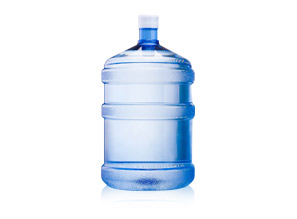# Water measuring riddle

582.8K Views

Using only a 5-gallon bucket and a 3-gallon bucket, put exactly four gallons of water in the 5-gallon bucket. (Assume you have an infinite supply of water. No measurement markings on the buckets.)take 3 gallon in 3 gallon put it in 5 gallon again 2 same 1 gallon will be left .empty 5 gallon put 1 gallon I n 5 gallon take 3 gallon in 3 gallon put it in 5 gallon.

(x,y) – state (x=0..5, y=0..3):
(5,0)
(2,3)
(2,0)
(0,2)
(5,2)
(4,3)

Fill the large bucket.  Pour 3 gallons of water into the small bucket and empty the remaining 2 gallons from the large bucket.  Pour the 3 gallons of water back into the large bucket.  Now completely fill the small bucket.  Fill the remaining 2 gallons in the large bucket with the water from the small bucket (there should be 1 gallon of water left in the small bucket).  Now empty the large bucket.  Take the 1 gallon of water from the small bucket and pour it in the large bucket.  Fill the small bucket completely and then dump that water into the large bucket.  Voila! There should now be 4 gallons of water in the 5-gallon bucket.